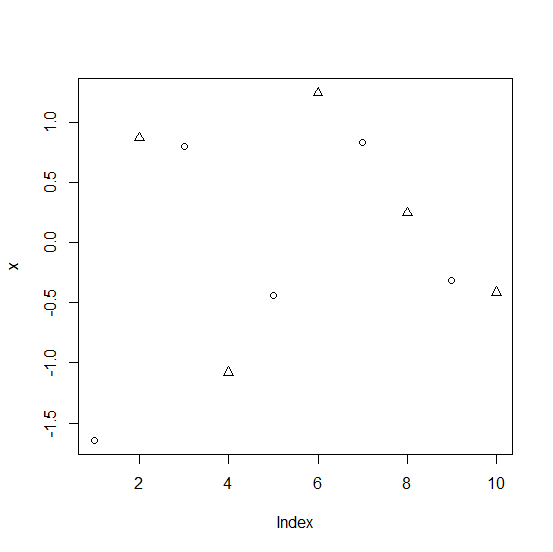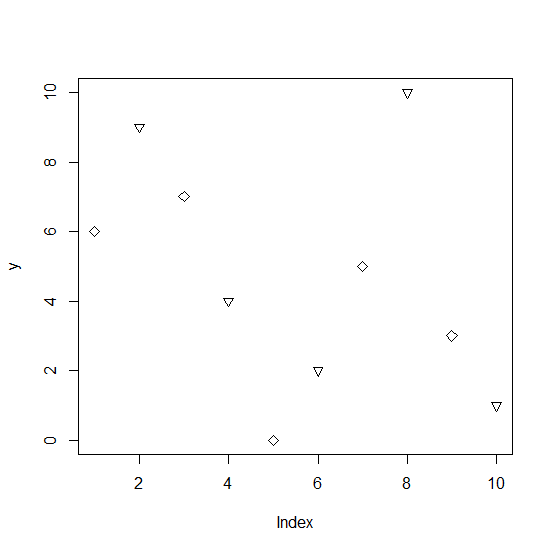# How to create a point chart in R with alternative points having different shape?

How to create a point chart in R with alternative points having different shape?

The pch argument is used to create different points in a base R chart and if we want to have alternative points of different shape then we can use rep function with pch argument but we need to use it for the exact length of the vector. For example, if we want to create a point chart of a vector that contains 10 values having different shapes of alternate points then we can use pch=rep(1:2,5)).

## Example1

Live Demo

> x<-rnorm(10)
> plot(x,pch=rep(1:2,5))

## Output:## Example

Let’s have a look at another example:

Live Demo

> y<-sample(0:10,10)
> plot(y,pch=rep(5:6,5))

## Output:Updated on: 06-Nov-2020

191 Views